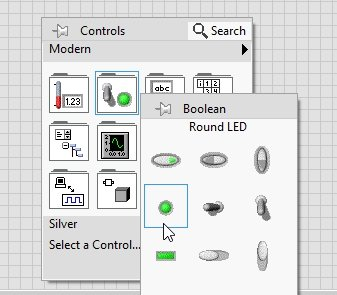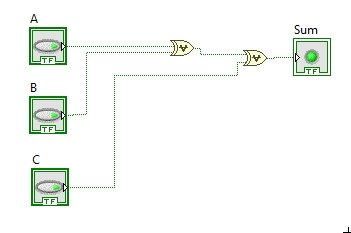# Design full adder circuit in labview : tutorial 32

In last tutorial we have seen how to design half adder circuit in labview and in this tutorial, you will learn how to design full adder circuit in labview. If you are familiar with digital logic design you must know what is the purpose and working of a full adder in digital logic design or digital systems. It is similar to the half adder we discussed in the previous tutorial. I will help you design a VI that will take to binary inputs from the user and ad the output return the sum and carry after adding them. At the start a brief introduction to full adder is provided after that a VI is designed which perform the full adder operation for three binary inputs. At the end of the tutorial you are provided with an exercise to do it by yourself.

### Design full adder circuit in labview

In order to add three binary digits we will need an adder namely a fulladder circuit. This adder will take three binary digits as input and at the output it will return two outputs names as sum and carry. Implementation of a full adder is a little more difficult than half adder as you will see shortly in the programming portion. A full-adder has three inputs and two outputs, where as a half adder has two inputs and two outputs this is the main difference between half adder and full adder.

### full adder circuit in labview Example

Figure 1: Boolean controls placement

Place three such push buttons for two binary single digit inputs. Now, in order to place indicator for the binary output indication we have to place LEDs. On the front panel click right and from the control palette select Boolean and then select Round LED as shown in the figure belowFigure 2: Boolean indicator

Figure 3: Control and indicators

Figure 4: Exclusive Or placement

• Place two such blocks as we are interested to do the XOR operation on three inputs. The output of these blocks will return the sum of the full adder output.
• At the input of the first block connect the two Boolean controls A and B and connect its output to the input of next XOR block and connect C control to the remaining input node of the second XOR gate, at the output of second XOR gate, connect the Boolean indicator i.e. the round LED you created and named as sum previously, as shown in the figure below,Figure 5: Summing block of full adder

Figure 6: And block placement

Figure 7: Or block placement

Figure 8: Carry block of full adder

Figure 9: Block diagram

• The output of the full adder should be according to the table given below,
 A B C Sum Carry 0 0 0 0 0 0 0 1 1 0 0 1 0 1 0 0 1 1 0 1 1 0 0 1 0 1 0 1 0 1 1 1 0 0 1 1 1 1 1 1

Figure 10: Output 1

Figure 11: Output 2

Exercise:

• Test the designed circuit for all the remaining combinations from the table and verify the output from the table.

<<Previous tutorial                                                                  Next tutorial>>